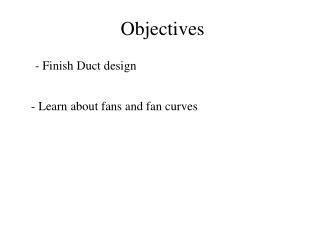# Objectives - PowerPoint PPT PresentationDownload PresentationObjectives

ObjectivesDownload Presentation## Objectives

- - - - - - - - - - - - - - - - - - - - - - - - - - - E N D - - - - - - - - - - - - - - - - - - - - - - - - - - -
##### Presentation Transcript

1. Objectives - Finish Duct design - Learn about fans and fan curves

2. Pressures • Static pressure • Velocity pressure • Total pressure – sum of the two above

3. Relationship Between Static and Total Pressure

4. Total and static pressure drops are proportional to square of velocity Plot of pressure drop vs. volumetric flow rate (or velocity) is called system characteristic Duct Design

5. System Characteristic

6. Electrical Resistance Analogy

7. Frictional Losses

8. Non-circular Ducts • Parallel concept to wetted perimeter

9. Dynamic losses • Losses associated with • Changes in velocity • Obstructions • Bends • Fittings and transitions • Two methods • Equivalent length and loss coefficients

10. Loss Coefficients ΔPt = CoPv,0

11. Example 18.7 • Determine total pressure drop from 0 to 4

12. Conversion Between Methods

13. Fans • Driving force to move air in buildings • Raise pressure and produce flow • Two main types: • Centrifugal • Axial

14. Relevant Fan Parameters • Total pressure rise • Static pressure rise • Power requirement • Efficiency • Note ηtot does not account for motor efficiency

15. Balance Point

16. Fan Performance

17. Centrifugal fans

18. Axial Fans

19. Fan Laws • “Approximate relationships” • Given two aerodynamically similar fans, can extrapolate performance data • Be very careful

20. Question • How does fan power consumption vary with volumetric air flow rate?

21. Controlling Air Flow

22. Dampers

23. Effect on Fan Curve

24. VAV Operation

25. Summary of Air Distribution Systems • Select diffuser based on ADPI • Given a layout of duct system • Calculate total pressure drop • Identify fittings contributing most to pressure drop • Deal with non-circular ducts • Use fitting loss coefficient or equal length method • Select the fan based on fan and duct curve